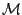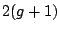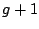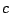# 4-edge-coloured graphs and 3-manifolds

Roman Nedela

Matej Bel University
Banská Bystrica, Slovakia

The homeomorphism problem on 3-manifolds attracts mathematicians since the beginnings of 20-th century. A particular but fundamental instance of it is known as the Poincare conjecture. Its central position in the core of classical mathematics is stressed by the fact that a formulation of the problem belongs to the list of the seven millennium problem proclaimed by the Clay Institute of Mathematics. The aim of the talk is to show how some classical mathematical problems can be formulated in a combinatorial way. One of the advantages of this approach is a possibility to use computer to study `small' examples. The most developed part of a combinatorial approach is knot theory based on a well-known fact that any 3-manifold can be constructed as a branched cover over the 3-sphere, where the set of branch points forms a knot. In our talk we present another, not so known combinatorial approach to 3-manifolds. We first introduce a combinatorial counterpart of the classical homeomorphism problem on 3-manifolds using a theory built by Pezzana and his successors. Pezzana proved that every closed compact orientable 3-manifoldcan be represented by a bipartite 4-edge-coloured 4-valent graph called a crystallisation of. Crystallisation is a 4-valent 4-edge-coloured graph such that removal of any monochromatic (perfect) matching yields a planar graph. This way 3-dimensional objects are replaced by 1-dimensional which allows us to employ combinatorial methods to study the homeomorphism problem. We explain a result of Casali and Grasselli showing that 3-manifolds of Heegaard genuscan be represented by crystallisations with a very simple structure which can be described by a-tuple of non-negative integers. The sum of firstintegers is called complexity of the admissible-tuple. Ifis the complexity then the number of vertices of the associated graph is. We give a combinatorial definition of the Heegaard genus and show how to classify 3-manifolds of Heegard at most one using graph theoretical results. Then we introduce an algorithm to derive a presentation of the fundamental group of a 3-manifold from the representation by means of a-tuple of integers. A 3-manifold is called prime if it cannot be expressed as a connected sum of non-trivial 3-manifolds. We show how to classify all prime 3-manifolds of Heegaard genus 2 described by 6-tuples of complexity at most 21. Finally, we add some remarks on the celebrated Thurston's geometrisation conjecture and on recent progress in the topic.

2005-05-23